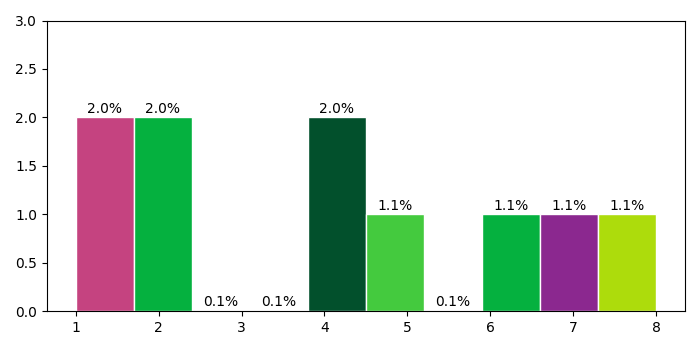# Plot a histogram with Y-axis as percentage in Matplotlib

To plot a histogram with Y-axis as percentage in matplotlib, we can take the following steps −

• Create a list of numbers as y.

• Create a number of bins.

• Plot a histogram using hist() method, where y, bins, and edgecolor are passed in the argument.Store the patches to set the percentage on Y-axis.

• Create a list of colors from the given alphanumeric numbers.

• To set the percentage, iterate the patches (obtained in step 3).

• Set the Y-axis ticks range.

• To display the figure, use show() method.

## Example

import random
import numpy as np
from matplotlib import pyplot as plt
plt.rcParams["figure.figsize"] = [7.00, 3.50]
plt.rcParams["figure.autolayout"] = True
y = [4, 1, 8, 7, 6, 4, 2, 1, 2, 5]
nbins = 10
_, _, patches = plt.hist(y, bins=nbins, edgecolor='white')
colors = ["#" + ''.join([random.choice('0123456789ABCDEF') for j in range(6)])
for i in range(nbins)]
for patch in patches:
patch.set_facecolor(colors[np.random.randint(100) % nbins])
x = patch.get_x() + patch.get_width() / 2
y = patch.get_height() + .05
plt.annotate('{:.1f}%'.format(y), (x, y), ha='center')
plt.ylim(0, 3)
plt.show()

## Output